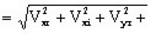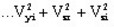# Modulus functions: ModV, ModC and Mod

## Before starting

The modulus functions, i.e. the vector modulus, the complex modulus and the general modulus, allow the computing of the modulus of scalar and vector quantities, expressed by real or complex numbers

Before describing the modulus functions, a recall of the notations is described in the following blocks.

## Scalar quantities: notations

To define the modulus functions of the scalar quantities expressed by real and complex numbers, we use the following notations:

• for a real scalar : A
• for a complex scalar : I

expressed by I = Ir + j Ii

where Ir and Ii are real scalars

## Vector quantities: notations

To define the modulus functions of the vector quantities expressed by real and complex numbers, we use the following notations:

• for a real vector : V

expressed by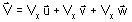where Vx , Vy and Vz , are real scalars

• for a complex vector : Vc

expressed by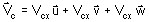where Vcx , Vcy and Vcz are complex scalars

It is also possible to describe a complex vector in the form:

where Vxr , Vyr , Vzr , Vxi , Vyi and Vzi are real scalars.

## Vector modulus: ModV function

The ModV (X) function returns the vector modulus of the X vector, which can be a real vector or a scalar vector.

• If V is a real vector, ModV(V) is a real scalar expressed by
$ModV\left(V\right)=\sqrt{Vx²+Vy²+Vz²}$
• If V c is a complex vector, ModV(V c ) is a complex scalar expressed by:

## Complex modulus: ModC function

The ModC (X) function returns the complex modulus of the complex argument X, which can be a complex scalar or a complex vector.

• If I is a complex scalar, ModC(I) is a real scalar expressed by: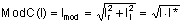• If Vc is a complex vector, ModC(Vc ) is a real vector expressed by:
$ModC\left(Vc\right)=\left(\begin{array}{c}ModC\left(Vcx\right)\\ ModC\left(Vcy\right)\\ ModC\left(Vcz\right)\end{array}\right)=\left(\begin{array}{c}\sqrt{Vxr²+Vxi²}\\ \sqrt{Vyr²+Vyi²}\\ \sqrt{Vzr²+Vzi²}\end{array}\right)$

## General modulus: Mod function

The Mod (X) function returns the general modulus of the argument X. Whatever is type of the argument X (scalar or vector, real or complex), the result Mod(X) is a real scalar.

• If I is a complex scalar, Mod(I) is a real scalar expressed by: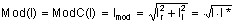• If V c is a complex vector, Mod(V c ) is a real scalar expressed by: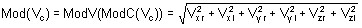## Overview

The results of the modulus functions (ModV, ModC and Mod) are described in the tables below.

Scalar

quantity

Vector modulus ModV Complex modulus ModC General modulus Mod

real scalar

A

no interest

complex scalar

I = Ir + j Ii

no interest

not authorized

real scalar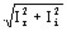real scalar

=Vector quantity Vector modulus ModV Complex modulus ModC General modulus Mod

real vector

V

real scalar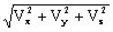no interest

returns Vr

real scalar

=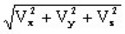complex vector

Vc = Vr + j Vi

complex scalar

= ModV (V r )

+ j ModV (V i )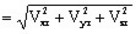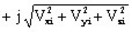real vector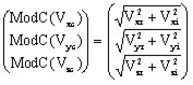real scalar

= ModV (ModC(Vc))